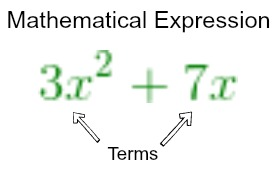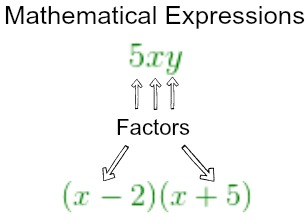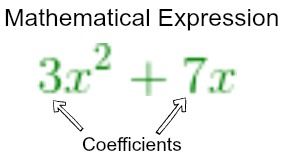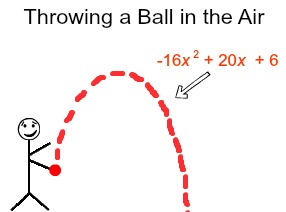# Parts of an Expression: Terms, Factors & Coefficients

Lesson Transcript
Instructor: Laura Pennington

Laura received her Master's degree in Pure Mathematics from Michigan State University, and her Bachelor's degree in Mathematics from Grand Valley State University. She has 20 years of experience teaching collegiate mathematics at various institutions.

In this lesson, we will look at some different parts that make up a mathematical expression. Those parts are terms, factors, and coefficients. We will define each of these and see what they look like in various examples.

## Parts of an Expression

Do you ever notice that the parts of a whole have their own individual labels and functions, but the whole couldn't function properly without even one of them? For instance, consider a baseball team. When a baseball team is on a field, there are nine different players that take up different positions on the field. There is a pitcher, a catcher, a first, second, and third baseman, a shortstop, and a left, right and center fielder in the outfield.

Each position on the field has its own label and its own function. When we put all these positions together, they work together to make up the whole, and if the team was missing a player at even one of these positions, the team's performance would suffer.

Surprisingly, this concept also applies to mathematical expressions! A mathematical expression is a phrase that contains numbers, variables, symbols and operators grouped together using addition, subtraction, multiplication, and division. For example, 3x 2 + 7x is a mathematical expression. Each mathematical expression has different parts to it, and just like the players on a baseball team, these parts have different labels and different functions. Let's take a look at some of these parts.

An error occurred trying to load this video.

Try refreshing the page, or contact customer support.

Coming up next: Rewriting Algebraic Expressions Using Structure

### You're on a roll. Keep up the good work!

Replay
Your next lesson will play in 10 seconds
• 0:01 Parts of an Expression
• 1:15 Terms, Factors, and…
• 3:15 Examples
• 4:57 Lesson Summary
Save Save

Want to watch this again later?

Log in or sign up to add this lesson to a Custom Course.

Timeline
Autoplay
Autoplay
Speed Speed

## Terms, Factors, and Coefficients

As we said, a mathematical expression contains numbers, variables, symbols, and operators connected by addition, subtraction, multiplication, and division. First, let's consider the parts of the expression that are connected with addition and subtraction. These parts are called terms.

Consider our example 3x 2 + 7x. We see that 3x 2 and 7x are connected by addition. Therefore, in this mathematical expression, we call 3x 2 and 7x terms.Next, let's talk about factors in a mathematical expression. In a mathematical expression, factors are parts of the expression that are connected by multiplication. For instance, consider the mathematical expressions 5xy and (x - 2)(x + 5). In the first expression, we see we are multiplying 5 by x by y, so we would call 5, x, and y factors of the mathematical expression. Similarly, in the expression (x - 2)(x + 5), we are multiplying (x - 2) and (x + 5), so we call (x - 2) and (x + 5) factors of the expression.So far, so good, right? Let's consider one more part of a mathematical expression. The coefficients. A coefficient is a number that is multiplied by a variable in a mathematical expression. To illustrate this, consider the terms of our initial example:

3x 2 + 7x. In each of the terms 3x 2 and 7x, x is a variable, and in both terms, we are multiplying a number by the variable. In the first term, 3x 2, 3 is being multiplied by the variable, so 3 is a coefficient. In the second term, 7x, 7 is being multiplied by the variable, so 7 is a coefficient.We see that terms, factors, and coefficients make up mathematical expressions. These parts of an expression each have their own label and function. The more we work with mathematical expressions, the more familiar we will become with these parts, so let's take a look at some examples to really drive this concept home (baseball pun intended).

## Examples

Suppose you throw a ball in the air, and the ball's height from the ground can be modeled using the mathematical expression -16x 2 + 20x + 6.To unlock this lesson you must be a Study.com Member.
Create your account

### Register to view this lesson

Are you a student or a teacher?

### Unlock Your Education

#### See for yourself why 30 million people use Study.com

##### Become a Study.com member and start learning now.
Back
What teachers are saying about Study.com
Create an account to start this course today
Used by over 30 million students worldwide